## Equal

Two quantities are said to be equal if they are, in some well-defined sense, equivalent. Equality of quantitiesand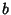is written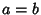.

A symbol with three horizontal line segments (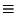) resembling the equals sign is used to denote both equality by definition (e.g.,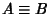meansis Defined to be equal to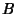) and Congruence (e.g.,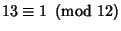means 13 divided by 12 leaves a Remainder of 1--a fact known to all readers of analog clocks).